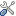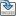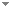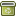# Boundedness and absoluteness of some dynamical invariants in model theory

Journal of Mathematical Logic 19 (2):1950012 (2019)

 Abstract Let [Formula: see text] be a monster model of an arbitrary theory [Formula: see text], let [Formula: see text] be any tuple of bounded length of elements of [Formula: see text], and let [Formula: see text] be an enumeration of all elements of [Formula: see text]. By [Formula: see text] we denote the compact space of all complete types over [Formula: see text] extending [Formula: see text], and [Formula: see text] is defined analogously. Then [Formula: see text] and [Formula: see text] are naturally [Formula: see text]-flows. We show that the Ellis groups of both these flows are of bounded size, providing an explicit bound on this size. Next, we prove that these Ellis groups do not depend on the choice of the monster model [Formula: see text]; thus, we say that these Ellis groups are absolute. We also study minimal left ideals of the Ellis semigroups of the flows [Formula: see text] and [Formula: see text]. We give an example of a NIP theory in which the minimal left ideals are of unbounded size. Then we show that in each of these two cases, boundedness of a minimal left ideal is an absolute property and that whenever such an ideal is bounded, then in some sense its isomorphism type is also absolute. Under the assumption that [Formula: see text] has NIP, we give characterizations of when a minimal left ideal of the Ellis semigroup of [Formula: see text] is bounded. Then we adapt the proof of Theorem 5.7 in Definably amenable NIP groups, J. Amer. Math. Soc. 31 609–641 to show that whenever such an ideal is bounded, a certain natural epimorphism 863–932]) from the Ellis group of the flow [Formula: see text] to the Kim–Pillay Galois group [Formula: see text] is an isomorphism. We also obtain some variants of these results, formulate some questions, and explain differences which occur when the flow [Formula: see text] is replaced by [Formula: see text]. Keywords No keywords specified (fix it) Categories (categorize this paper) DOI 10.1142/s0219061319500120 OptionsEdit this recordMark as duplicateExport citationFind it on ScholarRequest removal from indexRevision history

PhilArchive copy

Upload a copy of this paper     Check publisher's policy     Papers currently archived: 70,130

Setup an account with your affiliations in order to access resources via your University's proxy server
Configure custom proxy (use this if your affiliation does not provide a proxy)

## References found in this work BETA

Forking and Dividing in NTP₂ Theories.Artem Chernikov & Itay Kaplan - 2012 - Journal of Symbolic Logic 77 (1):1-20.
Hyperimaginaries and Automorphism Groups.D. Lascar & A. Pillay - 2001 - Journal of Symbolic Logic 66 (1):127-143.
Galois Groups of First Order Theories.E. Casanovas, D. Lascar, A. Pillay & M. Ziegler - 2001 - Journal of Mathematical Logic 1 (02):305-319.
Topological Dynamics of Definable Group Actions.Ludomir Newelski - 2009 - Journal of Symbolic Logic 74 (1):50-72.

## Similar books and articles

Invariants and Mathematical Structuralism.Georg Schiemer - 2014 - Philosophia Mathematica 22 (1):70-107.
Quantum Complementarity and Information Invariance.Časlav Brukner & Anton Zeilinger - 1999 - Vienna Circle Institute Yearbook 7:231-234.
On Some Dynamical Aspects of NIP Theories.Alireza Mofidi - 2018 - Archive for Mathematical Logic 57 (1-2):37-71.
Epistemic Boundedness.Andre Kukla - 1993 - International Studies in the Philosophy of Science 7 (2):121 – 126.
The Dynamical Model is a Perceptron.Bruce Bridgeman - 1998 - Behavioral and Brain Sciences 21 (5):631-632.
Forcing Absoluteness and Regularity Properties.Daisuke Ikegami - 2010 - Annals of Pure and Applied Logic 161 (7):879-894.
Making the Case for Causal Dynamical Triangulations.Joshua H. Cooperman - 2015 - Foundations of Physics 50 (11):1739-1755.
Getting Real About Invariants.Alan Costall, Giulia Parovel & Michele Sinico - 2001 - Behavioral and Brain Sciences 24 (2):219-220.